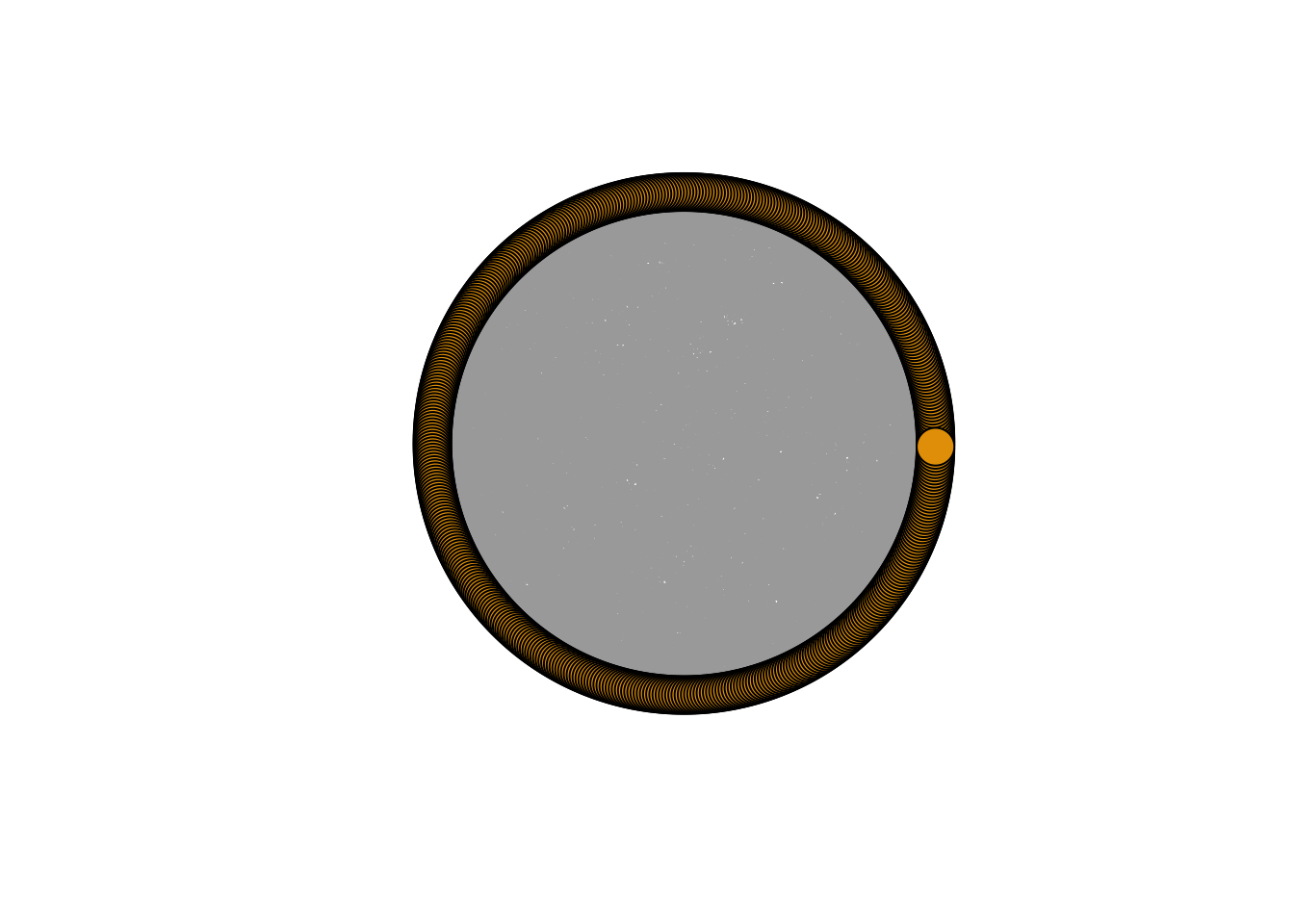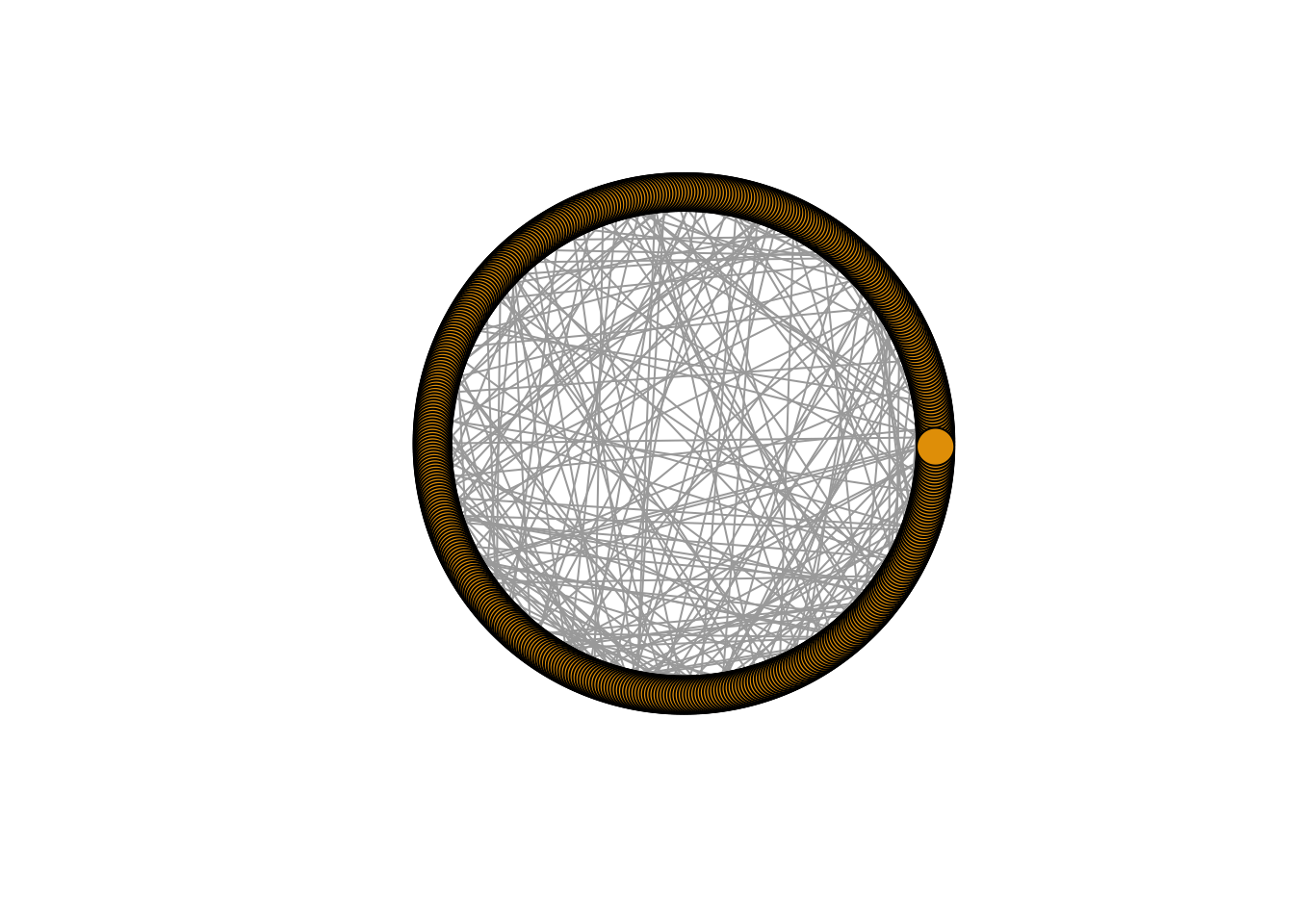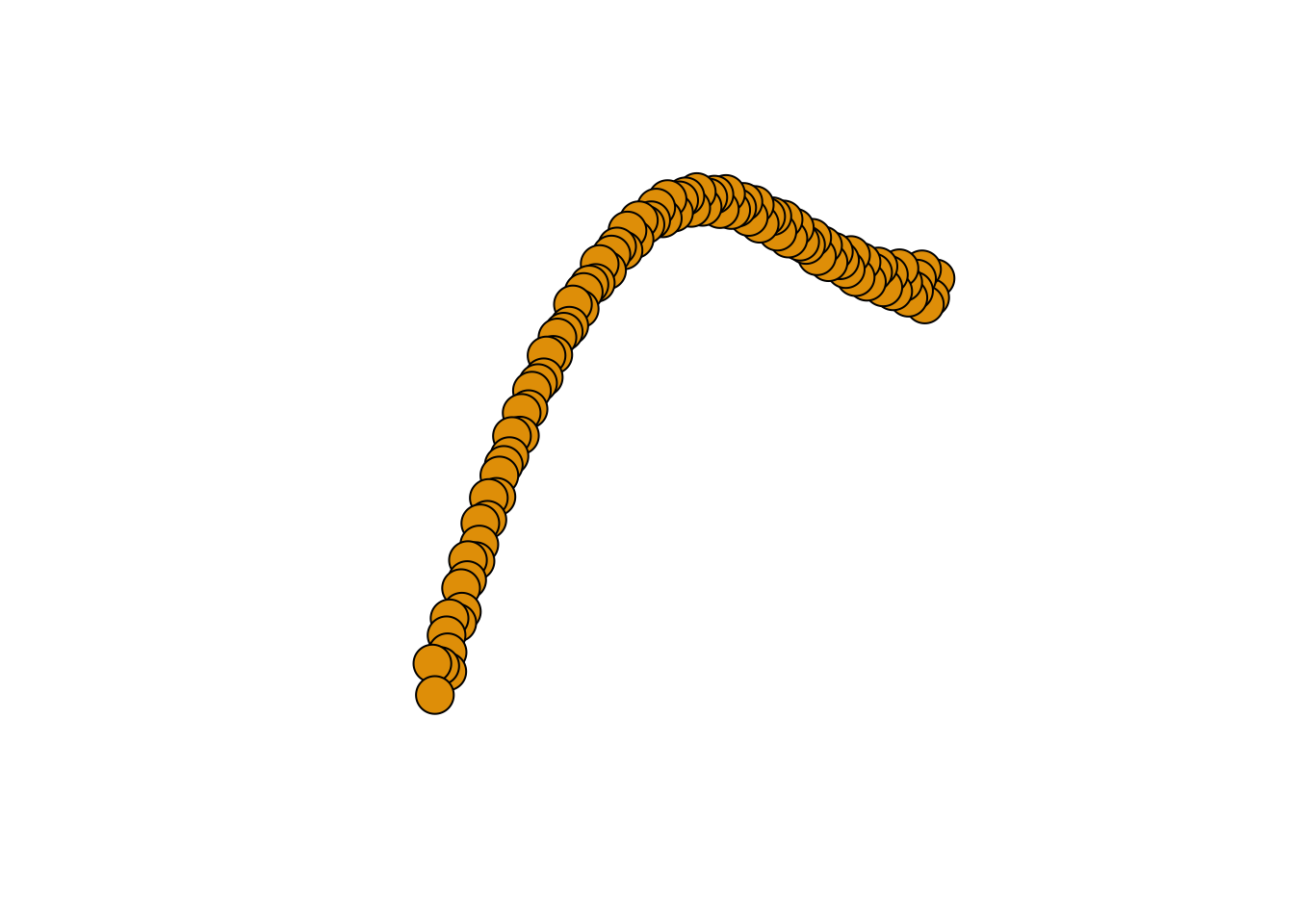Author

Emmanouil Tranos

Published

November 11, 2022

## Setup

Pay attention on the packages `sand` and `igraphdata`. The first one contains all the different network datasets used by . Then, the second one contain network data to be used together with the `igraph` package. More information can be found here and here. Feel free to explore these network data on your own time.

``````library(tidyverse)
library(igraph)

library(sand)
library(igraphdata)``````

## Aims of the practical

• Simulate network models

• Analyse their key attributes

• Compare them

`igraph` enables us to generate networks based on some of the some key network models we discussed. I list below some of these functions:

• `sample_gnp()`

• `sample_smallworld()`

• `sample_pa()`

Go to `igraph`’s webpage and familiarise yourselves with the syntax of these functions.

Task: Create three networks using these three functions with, let’s say, (n = 500) nodes each.

Visualise and compare these networks.

Importantly, just before you generate a network with one of the above three functions you will need to define a random seed for reproducibility reasons, e.g. `set.seed(55)`.

Question: Without defining a random seed, generate a network as per the above, run some type of quick analysis about this network and then repeat the code by generating a second network with the exact same characteristics. Run the same analysis for the second network. Are the results the same?

Why do we need to define the random seed ?

## Examples of modelled networks

``````sample_gnp(n = 500, p = 0.02) %>%
plot(layout=layout_in_circle, vertex.label=NA)````````````sample_pa(n = 500, directed=FALSE) %>%
plot(layout=layout_in_circle, vertex.label=NA)````````````sample_smallworld(dim = 1, size = 500, nei = 5, p = 0.05) %>%
plot(layout=layout_in_circle, vertex.label=NA)````````````make_lattice(dim =1, length = 100, nei = 5) %>%
plot(vertex.label=NA)``````## References

Kolaczyk, Eric D, and Gábor Csárdi. 2020. Statistical Analysis of Network Data with r. Second. Vol. 65. Springer.